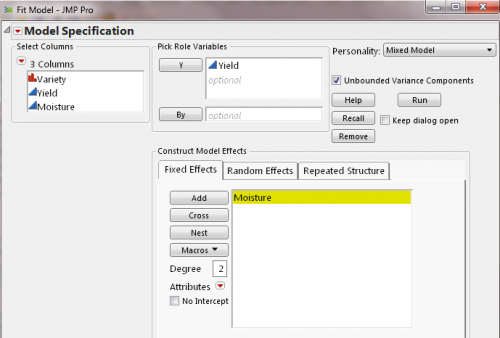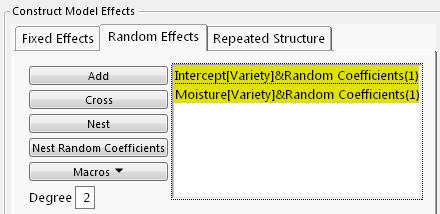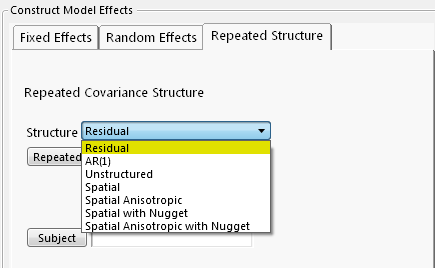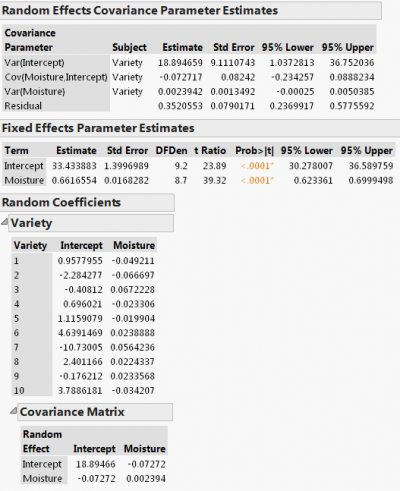Our World Statistics Day conversations have been a great reminder of how much statistics can inform our lives. Do you have an example of how statistics has made a difference in your life? Share your story with the Community!
Choose Language Hide Translation Bar
JMP Pro for linear mixed models — Part 1

JMP Pro 11 has added a new modeling personality, Mixed Model, to its Fit Model platform. What’s a mixed model? How does JMP Pro fit such a model? What are the key applications where mixed models can be applied? In this and future blog posts, I will try to dispel myths about mixed models and illustrate the software’s capabilities with real-life examples.

What’s a Linear Mixed Model?

Linear mixed models are a generalization of linear regression models, y=Xβ+ε . This model is fit to a sample of cross-sectional data by standard least squares to estimate the fixed-effect parameters, β. Extending the model to allow for random effects, Z, the new regression model becomes y=Xβ+Zγ+ε. It’s called the mixed model because there are both fixed effects and random effects.

We make the following assumptions about random effect parameters, γ and random error ε : (1) γ and ε are normally distributed, and (2) there are no covariance between γ and ε. JMP provides an unstructured covariance structure for γ, and several commonly used structures for ε. Using the restricted maximum likelihood method (REML), JMP jointly estimates β as well as covariance matrices for γ and ε . In order to fit such a model, additional data on each subject is required, or, in case of modeling spatial data, dimensions of measurements are needed. (In recent years, mixed model theory has been extended to encompass such statistical methods as empirical Bayes, ridge regression, time series and smoothing splines. However, I limit the scope of my discussion to the “traditional” use of linear mixed models.)

Why Mixed Models?

When there exists correlation between responses or an important causal factor is omitted, failure to account for that leads to under- or overestimating the effects of treatment and other factors.

Here are some of common use cases for mixed models:

• Allowing coefficients (e.g., intercept and slope) to vary randomly across subjects (random coefficient models). A variant is the individual growth model, which can be applied to predict individual growth trajectory and degradation data analysis.
• Analysis of randomized block designs, and split-plot designs where hard-to-change and easy-to-change factors result in multiple error terms.
• Controlling for unobserved individual heterogeneity in the form of random effects (panel data models).
• Analysis of repeated measures where within-subject errors are correlated.
• Correlated responses where different measures are taken from the same subjects.
• Subjects are hierarchical (e.g., students within schools). This is known as a hierarchical linear model or multilevel model.
• Spatial variability (geostatistics).
• The list goes on and on. With JMP Pro 11, you can easily specify and fit all of these models using the point-and-click interface and review the results in a user-friendly way. Before I turn to my first example, let me outline the general steps for specifying your mixed model in JMP Pro.

Steps for Specifying Mixed Models

1. Select Analyze =>Fit Model, and choose Mixed Model Personality.
2. Select a continuous response variable as Y and construct fixed effects as you normally would do with a standard least squares fit.
3. Use the Random Effects tab to specify random coefficients or random effects.
4. Use the Repeated Structure tab to select a covariance structure for model errors.
5. Click Run.
6. Example 1: Random Coefficient Models — Allowing Coefficients to Vary Randomly Across Subjects

In this example, we’re interested in estimating the effect on wheat yield of pre-planting moisture in the soil while allowing each variety to have random deviation from population effects. So, a random coefficient model is called for. The experiment randomly selects 10 varieties from the wheat population and assigns each to six one-acre plots of land. In total, 60 observations with six measurements of yield for each variety are collected. (The data, “Wheat,” is available in JMP’s Sample Data folder.)

I followed the steps laid out above to specify my random coefficient model. From the Fixed Effects tab, I added fixed effects (i.e., population intercept and population Moisture effect).From the Random Effects tab, I used the Nest Random Coefficients button to specify that a variety’s intercept and Moisture effect vary randomly from one to another. Note that JMP’s covariance structure for random coefficients is unstructured.From the Repeated Structure tab, I selected Residual for the model error term.This example is detailed in the JMP documentation. Let's examine the results. First, take a look at the Random Effects Covariance Parameter Estimates report.The variance estimate for Intercept is 18.89 with a standard error estimate of 9.11, so the z-score is 2.07 (=18.89/9.11). Using the Normal Distribution function from JMP Formula Editor (or look up in a standard normal distribution table in any statistics text book), we can find the p-value to be 0.0192, indicating that the variation in baseline yield (i.e., without any pre-planting watering) across varieties is statistically significant. Similarly, we obtain the-p-value for Cov(Moisture, Intercept), 0.3777, and p-value forVar(Moisture), 0.0380. Although the sign on the covariance estimate is negative, there is no statistical evidence that this negative correlation is significant. The variation in Yield across different moisture levels is significant at α=0.05.

The Random Coefficients report gives the BLUP (Best Linear Unbiased Predictor) values for how each variety is different from the population intercept and population Moisture effect (reported in Fixed Effects Parameter Estimates). For Variety 1, the estimated moisture effect on its yield is 0.61 (=0.66-0.05), baseline yield is 34.39 (=33.43+0.96), and the predicted yield equation is Yield=34.39+0.61*Moisture.

Combining both the fixed effects and random coefficient estimates, we find a significant overall effect on wheat yield of moisture and discover significant variation in the moisture effect across different varieties. The random coefficient model produces a BLUP prediction equation for yield for each variety.

Other Specifications of Random Coefficient Models

Individual Growth Model is a type of random coefficient model in which random time effect is estimated for each individual. After adding a continuous time variable (e.g., day, month, etc.) as a random effect, use the Nest Random Coefficients button to request a separate slope and intercept for each individual.

In education research, subjects are often nested in a hierarchical order. By adding multiple groups of random effect statements you can fit hierarchical linear models/multilevel models.

Stay tuned. In my next blog post, I will discuss using mixed models for panel data, repeated measures and spatial regression.

Article Labels

There are no labels assigned to this post.

Article Tags
Visitor

Walter Paczkowski wrote:

Great blog post. I'm very interested in how I can use this in consumer choice modeling. Specifically right now for linear models (just plain OLS) where each consumer rates a product on a 1 - 10 scale and does so for, say, 12 products, each varying by four factors (e.g., price, form, flavor, taste). I want to estimate a model allowing for the heterogeneity of each consumer. Is this possible with this personality?

WaltStaff

Jian Cao wrote:

Linear mixed model is not appropriate for modeling consumer choices as (1) ratings is a discrete ordinal variable and (2) explanatory variables are product-specific attributes.

Visitor

Walter Paczkowski wrote:

It's actually hotly debated whether the ratings are discrete or represent just points on a continuous, but latent, scale. Most who work with consumer data treat the ratings as continuous and use OLS for estimation. I'm in this camp. Regarding the explanatory variables, I can see your point -- so for mixed models, we need variables that are not product specific.

Visitor

Michael Bailey wrote:

I'm trying to work through this example, and I cannot get access to the wheat.jmp data. (My university has a site license to JMP 11.) Can anyone tell me where to get it?

Relatedly, in the other data file I'm trying to work through this example (Random Coefficients Model) with, I can't figure out how to use the "Nest Random Coefficients" button to get the two effects (e.g. Intercept[Variety]&Random Coefficients(1)) in the example above.Staff

Jian Cao wrote:

1. The data, wheat.jmp, is in the Sample Data Directory found at the JMP software main menu Help > Sample Data.

2. To specify a random coefficient model: (i) select the Random Effects tab, (ii) Select Moisture and click Add, (iii) Select Variety from the Select Columns list, select Moisture from the Random Effects tab, and then click Nest Random Coefficients.

You need to have JMP Pro 11 in order to run Mixed Models.

Visitor

P wrote:

I use JMP pro 11 and cant find the mixed model personality. Instead I can set factors as fixed or random when I define the model. Including random factors gives REML, but I cant specify structures such as poisson. My data is from counts and highly non-normal and I realy would like to run a mixed model with poisson distribution. Is this possible? And why cant I find the mixed model personality? Thankful for any insightsStaff

Jian Cao wrote:

You can launch Mixed Model by going to Analyze =>Fit Model platform. Make sure you are running JMP Pro 11 or later Mixed models in JMP Pro assumes a continuous response variable, so it is not appropriate for count data.

Level I

Hi, this post is very useful. One question, what if we have more than 1 variable to add as independent in the regression? Can we still use this methodology to understand the diferent slopes between population for more than 1 variable?

Thanks.